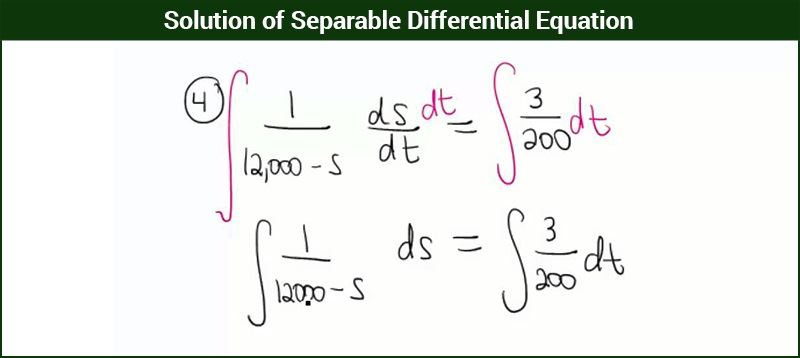# Solution Of Separable Differential Equation

A differential equation is an equation of the form

$$\begin{array}{l}\frac{\mathrm{d} y}{\mathrm{d} x} = g(x),\;\;\;\; where\;\; y = f(x)\end{array}$$
.

General equations involve Dependent and Independent variables, but those equation which involves variables as well as derivative of dependent variable (y) with respect to independent variable (x) are known as Differential Equation.

## Solving A Separable Differential Equation

The solution of a differential equation is a function, that represents a relationship between the variables, independent of derivatives.

Such as:

Given differential equation :

$$\begin{array}{l}\frac{\mathrm{d} y}{\mathrm{d} x} = \cos x\end{array}$$

Solution :

$$\begin{array}{l}y = \sin x + c\end{array}$$
(thus derivative is eliminated)

The solution of a differential equation is also known as its primitive. In the upcoming discussions, we will find out the solution of first order and first degree differential equations.

Generally, there are three methods to solve first order and first degree differential equation. We will be discussing only solution of differential equations with separation of variables.### Variable Separable Differential Equations

The differential equations which are expressed in terms of (x,y) such that, the x-terms and y-terms can be separated to different sides of the equation (including delta terms). Thus each variable separated can be integrated easily to form the solution of differential equation.

The equations can be written as

$$\begin{array}{l}f(x)dx + g(y)dy = 0\end{array}$$

where

$$\begin{array}{l} f(x) \end{array}$$
and
$$\begin{array}{l} g(y) \end{array}$$
are either constants or functions of
$$\begin{array}{l} x \end{array}$$
and
$$\begin{array}{l} y \end{array}$$
respectively.

In simpler terms all the differential equations in which all the terms involving

$$\begin{array}{l} x ~and~ dx \end{array}$$
can be written on one side of the equation and the terms involving
$$\begin{array}{l} y \end{array}$$
and
$$\begin{array}{l} dy \end{array}$$
on the other side are known as variable separable differential equations.

### Examples

 Lets Work Out- Example – Solve $$\begin{array}{l} \frac{dy}{dx} = \frac{x^3 + 3}{y^2 + 1}\end{array}$$. Solution – $$\begin{array}{l}(y^2 + 1)dy = (x^3 + 3)dx\end{array}$$ Integrating both the sides we get, $$\begin{array}{l}\int (y^2 + 1)dy = \int(x^3 + 3)dx\end{array}$$ $$\begin{array}{l}\frac{y^3}{3} + y = \frac{x^4}{4} + 3x + c\end{array}$$ $$\begin{array}{l}\frac{y^3}{3} + y – \frac{x^4}{4} – 3x = c\end{array}$$ It is the required solution. Example- Arjun is riding his bike at an initial velocity of 10 m/s.  To reach his home at time he continuously increases his velocity at the rate of $$\begin{array}{l} 10% \end{array}$$ in what time will his velocity be 2.718 times of what it is now? Solution- Let the velocity of Arjun be v at any time t. Then $$\begin{array}{l}\frac{dv}{dt} = \frac{10}{100} × V\end{array}$$ $$\begin{array}{l}\frac{dv}{dt} = \frac{V}{10}\end{array}$$ Separating the variables we get $$\begin{array}{l} \frac{1}{V}dv = \frac{1}{10}dt \end{array}$$ Integrating both the sides we get; $$\begin{array}{l} ln V = \frac{t}{10} + c \end{array}$$ Where c is any arbitrary constant; $$\begin{array}{l} V = e^{\frac{t}{10}} × e^{c} \end{array}$$ $$\begin{array}{l}V = Ce^{\frac{t}{10}} \end{array}$$ We know at $$\begin{array}{l} t = 0, V = 10 m/s\end{array}$$ So the equation becomes V = $$\begin{array}{l} 10e^{\frac{t}{10}}\end{array}$$ Now $$\begin{array}{l} V = 2.718 × 10 \frac{m}{s}\end{array}$$ Substituting this value we get, $$\begin{array}{l} 27.18 = 10 e^{\frac{t}{10}} \end{array}$$ $$\begin{array}{l} \Rightarrow e^{\frac{t}{10}} = 2.718 \end{array}$$ $$\begin{array}{l} \Rightarrow ln e^{\frac{t}{10}} = ln 2.718 \end{array}$$ $$\begin{array}{l} \Rightarrow \frac{t}{10} = 1\end{array}$$ $$\begin{array}{l} \Rightarrow t = 10 seconds \end{array}$$

### Solving Exact Differential Equations

In the equation

$$\begin{array}{l} f(x)dx + g(y)dy = 0 \end{array}$$

If

$$\begin{array}{l} \frac{\partial d f(x)}{\partial dx}\end{array}$$
=
$$\begin{array}{l}\frac{\partial d g(y)}{\partial dx}\end{array}$$
then the equation is called as exact differential equation. In the first integral variable y is taken as a constant and in the second integral variable x is taken as constant.

Thus the solution is given by

$$\begin{array}{l} \int f(x) dx + \int g(y) dy = c \end{array}$$

 Lets Work Out- Example- Solve the following :- $$\begin{array}{l} (x^3 + 3xy^2 + 25)dx + (y^3 + 3yx^2 + 25)dy = 0 \end{array}$$ Solution- $$\begin{array}{l}\frac{\partial d f(x)}{\partial dx} = 3x^2 + 3y^2\end{array}$$ $$\begin{array}{l}\frac{\partial d g(y)}{\partial dx} = 3x^2 + 3y^2\end{array}$$ Since$$\begin{array}{l} \frac{\partial d f(x)}{\partial dx} = \frac{\partial d g(y)}{\partial dx}\end{array}$$ , it is an exact differential equation. Its solution is $$\begin{array}{l}\int f(x)dx + \int g(y)dy = c \end{array}$$ ⇒ $$\begin{array}{l}c = \int(x^3 + 3xy^2 + 25)dx + \int(y^3 + 25)dy\end{array}$$ ⇒ $$\begin{array}{l} c = \frac{x^4}{4} + \frac{y^4}{4} + \frac{3}{2}x^2y^2 + 25(x + y)\end{array}$$ ⇒ $$\begin{array}{l} c = x^4 + y^4 +6 x^2 y^2 +100(x + y)\end{array}$$

Stay with us to explore more and more on differential equations. Learn other methods to solve differential equations. Download the BYJU’s learning app to keep learning.

Quiz on Solution of Separable Differential Equation